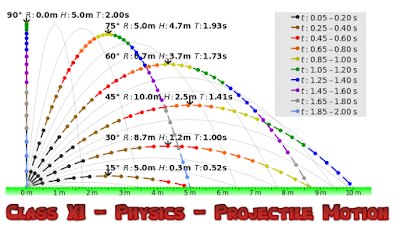## Class XI - PHYSICS - PROJECTILE MOTION - (Assignment)image credits: cmglee (Wikimedia)

1. An aeroplane is flying in horizontal direction with a velocity of 600 km/h and at a height of 1960 m. When it is vertically above the point A on the ground, a body is dropped from it. The body strikes the ground at a point B. Calculate distance AB.

2. A body is thrown horizontally from the top of a lower and strike the ground in 3 seconds at an angle 45° with the horizontal. Find the height of the lower and the speed with which it was projected.
[Answer:  44.1 m , 29.4 m/s ]

3. An air craft is flying at a height of 3,500 m above the ground. If the angle subtended at a ground observation point by the air craft positions 10 s apart is 30° while passing over his head, what is the speed of the air craft?

4. A ball is thrown horizontally from the top of a lower with a speed of 50 m/s. Find the velocity and position at the end of 3 second g = 9.8 ms⁻².
[Answer: 58 m/s 30° 27' with horizontal ]

5. A ball is projected from ground with a velocity of 19.6 m/s at an angle of 30 0 with the horizontal. Determine its time of flight and the horizontal distance it travels.

6. Prove that the maximum horizontal range is 4 times the maximum height attained by a projectile which is fired along the required oblique direction.

7. Find the minimum velocity with which the horizontal range is 39.2 m.

8. The ceiling of a long hall is 25 m high. What is the maximum horizontal distance that the ball thrown with a speed of 40 m/s can go without hitting the ceiling of the hall.

9. Show that the maximum range of a projectile in any direction is described in the same time in which it would fall freely under gravity through this distance starting from rest.

10. A target is fixed the top of a pole 13 m high. A person standing at distance 50 m from the pole is capable of projecting a stone with a velocity 10√g m/s. If he wants to strike the target in shortest possible time at what angle should be project the stone.

11. A projectile is projected at time t = 0 , from a point P on the ground with a speed v₀ at an angle 45° to the horizontal. Find the angular momentum of the particle about the point P at time t = v₀/g.

12. A grass hopper can jump a maximum horizontal distance of 20.4 cm. If it spends negligible time on the ground, what is its speed of travel along the road (Take g = 10 m/s²).

13. A body is projected such that its kinetic energy at the top is 3/4th of its initial kinetic energy. What is the initial angle of projection of the projectile with the horizontal?

14. A hunter aims his gun and fires a bullet leaves the barrel of the gun, the monkey dropped. Will the bullet hit the monkey? Substantiate your answer with proper reasoning.

15. A cricketer can throw a ball to a maximum horizontal distance of 100 m. With the same speed how high above the ground can the cricket throw the same ball.

16. Calculate the angular velocity of the minute’s hand of a clock.

17. A body is moving in a circle of radius 100 cm with a time period of 2 second. Find the acceleration.

18. A cyclist is riding with a speed of 27 km/h. As he approaches a circular turn of the road of radius 80.0 m, he applies brakes and reduces his speed at a constant rate of 0.5 m/s per second. Find the magnitude of the net acceleration of the cyclist.